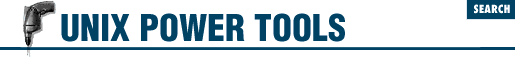#Chapter 49# 49. Working with Numbers

## 49.1 bc: Simple Math at the Shell Prompt

Want to do a few simple calculations? Standard UNIX provides two simple calculators: dc (desk calculator) and bc. (Who knows what the b stands for? The manual page refers to it only as "an arbitrary precision arithmetic language.")

To a novice, dc sounds more promising. However, it's a reverse-Polish calculator. You enter each operand on a separate line, followed by an operator. The operands are stored on a stack; the operator pops them from the stack, replacing them with the result. Unfortunately for the novice, the result isn't printed. You need to type p ("print") to get any output.

bc is actually much easier to use:

```% ```bc
5*2```
10
[CTRL-d]
%```

Simply type an arithmetic expression, followed by a RETURN. The result will be printed to standard output. Type CTRL-d to exit.

The only thing you need to learn to find bc really useful is the scale command, which tells the calculator how many decimal places to use. The default is 0, so typing an expression like `10/4` yields the unfortunate answer 2. However:

```% ```bc
scale=2
10/4```
2.50```

gives a more acceptable two decimal places. Scale can be set from 0 to 99 decimal places.

As an alternative, invoke bc with the -l option, which will automatically give you up to 20 decimal places worth of precision (but a lot of trailing zeros on simple division).

bc is really quite complete - you can even define your own functions. See the bc manual page for details. It's also useful for base conversion (49.2).

expr can also be used to do simple math (49.6) on the command line, but it's really better suited for doing math in shell scripts (45.28).

Of course, if you're running the X Window System (1.31), you can just use xcalc, which draws a Texas Instruments or HP calculator right on the screen, and lets you punch keys on the keyboard or with the mouse.

- TOR48.12 Using index with a Filter49.2 bc: Hexadecimal or Binary Conversion